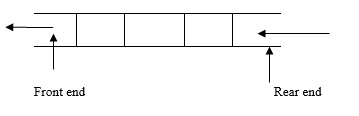# Explain deleting an element in a queue by using C language

Data structure is collection of data organized in a structured way. It is divided into two types as explained below −

• Linear data structure − Data is organized in a linear fashion. For example, arrays, structures, stacks, queues, linked lists.

• Nonlinear data structure − Data is organized in a hierarchical way. For example, Trees, graphs, sets, tables.

## Queue

It is a linear data structure, where the insertion is done at rear end and the deletion is done at the front end.The order of queue is FIFO – First In First Out

### Operations

• Insert – Inserting an element into a queue.
• Delete – Deleting an element from the queue.

### Conditions

• Queue over flow − Trying to insert an element into a full queue.

• Queue under flow − Trying to delete an element from an empty queue.

## Algorithms

Given below is an algorithm for insertion ( ) −

• Check for queue overflow.
if (r==n)
printf ("Queue overflow")
• Otherwise, insert an element in to the queue.
q[r] = item
r++

Given below is an algorithm for deletion ( )

• Check for queue under flow.
if (f==r)
printf ("Queue under flow")
• Otherwise, delete an element from the queue.
item = q[f]
f++

Given below is an algorithm for display ( )

• Check whether the queue is empty or not.
if (f==r)
printf("Queue is empty")
• Otherwise, print all the elements from ‘f’ to ‘r’.
for(i=f; i<r; i++)
printf ("%d", q[i]);

## Program

Following is the C program for deleting an element in queue −

#include <stdio.h>
#define MAX 50
void insert();
int array[MAX];
int rear = - 1;
int front = - 1;
main(){
int choice;
while (1){
printf("1.Insert element to queue ");
printf("2.Delete an element from queue");
printf("3.Display elements of queue ");
printf("4.Quit ");
scanf("%d", &choice);
switch (choice){
case 1:
insert();
break;
case 2:
delete();
case 3:
display();
break;
case 4:
exit(1);
default:
printf("Wrong choice ");
}
}
}
void insert(){
if (rear == MAX - 1)
printf("Queue Overflow ");
else{
if (front == - 1)
/*If queue is initially empty */
front = 0;
printf("Inset the element in queue : ");
rear = rear + 1;
}
}
void display(){
int i;
if (front == - 1)
printf("Queue is empty ");
else{
printf("Queue is : ");
for (i = front; i <= rear; i++)
printf("%d ", array[i]);
printf("");
}
}
void delete(){
if (front == - 1 || front > rear){
printf("Queue Underflow ");
return ;
}
else{
printf("Element deleted from queue is : %d",array[front]);
front = front + 1;
}
}

## Output

When the above program is executed, it produces the following result −

1.Insert element to queue
2.Delete an element from queue
3.Display elements of queue
4.Quit
Inset the element in queue: 12
1.Insert element to queue
2.Delete an element from queue
3.Display elements of queue
4.Quit
Inset the element in queue: 23
1.Insert element to queue
2.Delete an element from queue
3.Display elements of queue
4.Quit
Inset the element in queue: 34
1.Insert element to queue
2.Delete an element from queue
3.Display elements of queue
4.Quit
Element deleted from queue is: 12
Queue is:
23 34
1.Insert element to queue
2.Delete an element from queue
3.Display elements of queue
4.Quit
Enter your choice: 4